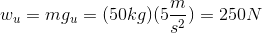# AP Physics C: Mechanics : Calculating Weight

## Example Questions

### Example Question #1 : Calculating Weight

The force on an object due to gravity on the moon is one-sixth of that found on Earth. What is the acceleration due to gravity on the moon?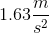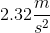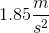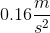Explanation:

We can use Newton's second law: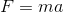Set up equations for the force on the moon and the force on Earth: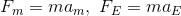Now we can use substitution: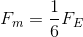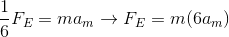From this, we can see that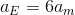. Using the acceleration due to gravity on Earth, we can find the acceleration due to gravity on the moon.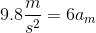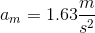### Example Question #2 : Calculating Weight

A large planet exerts a gravitational force five times stronger than that experienced on the surface of Earth. What is the weight of a 50kg object on this planet?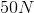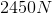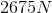Explanation:

The weight of the object on Earth's surface is: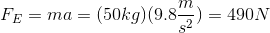The force on the new planet is five times that on Earth, so we can simply multiply: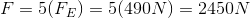### Example Question #1 : Calculating Weight

A space woman finds herself in an unkown planet with gravity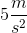. If her weight on Earth is 500N, what is her weight on the unkown planet?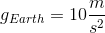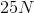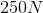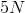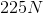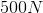Explanation:

We know that the weight of an object is given by: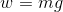is the mass of the object and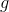is the gravitational acceleration of whatever planet the object happens to be on.

We know the gravity on the unkown planet, so the weight of the woman is given by: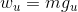We need only to find the mass of the woman to solve the problem. Since the mass of the woman is constant, we can use the information about her weight on Earth to figure out her mass.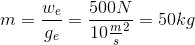Use this mass to solve for her weight on the new planet.